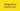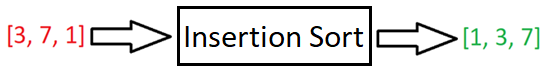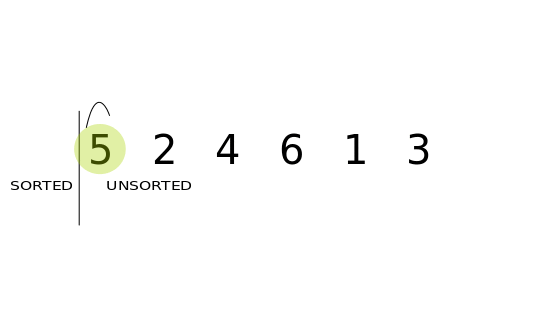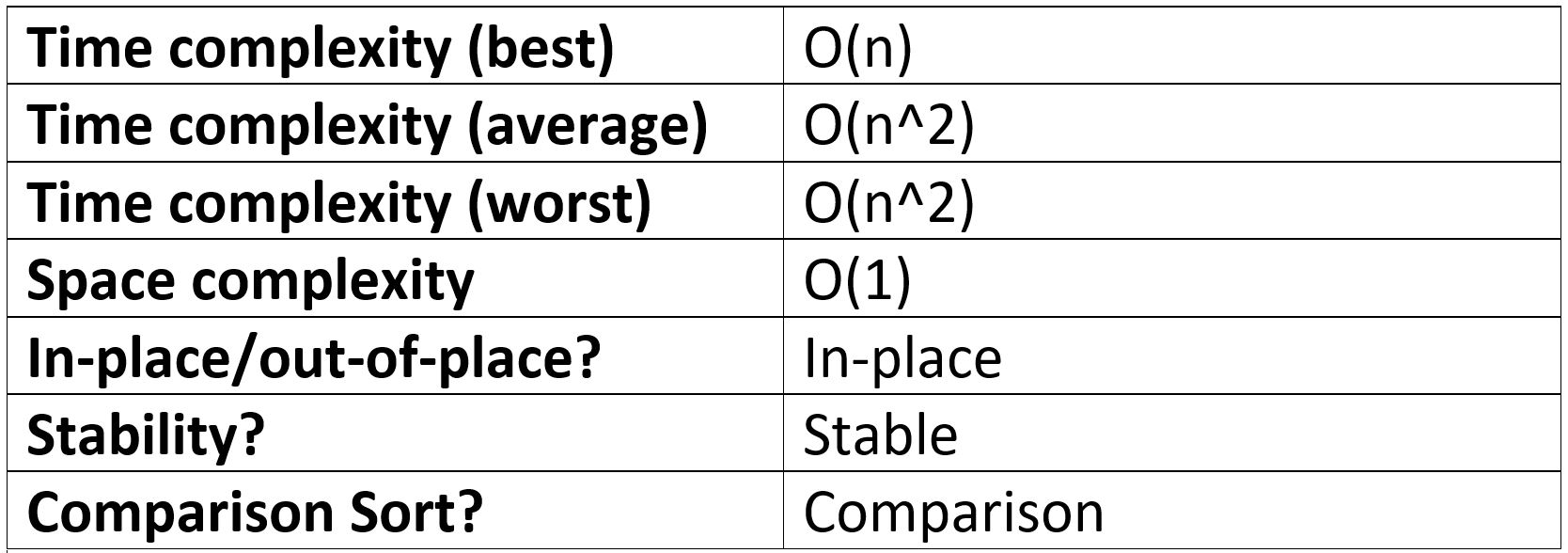# Insertion Sort in JavaScript

-26 July 2021## What is the Insertion Sort Algorithm?

Insertion sort is one of the more intuitive sorting algorithms. Despite it being less efficient than algorithms such as Merge Sort and Quick Sort, it is able to outperform them in certain use cases.

Insertion Sort takes an array, puts it in order, and spits it out:Insertion Sort is a stable, in-place, and comparison-type algorithm.

Stable means that two elements with equal values will appear in the same order in the sorted output as they appear in the unsorted input array.

For example, if we wanted to sort:

`[“Cherries“, “Blackberries”, “Apples”, “Bananas”]`

into alphabetical order by first letter, the output would be:

`[“Apples”, “Blackberries”, “Bananas”, “Cherries”]`

As you can see, “Blackberries” and “Bananas” remained in the same relative positions in the input and output array because the algorithm is stable. Bubble Sort, Merge Sort, and Radix Sort are also stable sorting algorithms.

If the algorithm was unstable, then “Bananas” and “Blackberries” may be interchanged. Selection Sort, Heap Sort and Quick Sort are examples of unstable sorting algorithms.

For a good and simple example of when it’s important to know whether an algorithm is stable or not, check out this article: Important Algorithm Concepts | Algorithm Stability, In-place Algorithms, and Comparison Algorithms

What’s an in-place algorithm? Here’s Wikipedia’s answer: “an algorithm which transforms input using no auxiliary data structure. However, a small amount of extra storage space is allowed for auxiliary variables.”

In simple terms, it usually just means that the input is overwritten (via swapping or replacement) by the output as the algorithm executes. The advantage of in-place algorithms is that they take up less space in memory.

And finally, a comparison sort is a sorting algorithm that only reads the list of elements through a single abstract comparison operation (usually a “less than” or “equal to”) that determines which of the two elements should occur first in the final sorted output array.

## Insertion Sort step-by-step

Insertion Sort works by comparing an element with the elements to its left, until it reaches an element that is smaller than it; the element is then inserted in front of the smaller element.The gif above shows how Insertion Sort works. Let’s go through Insertion Sort step-by-step:

1. Pass the unsorted array [5, 2, 4, 6, 1, 3] into Insertion Sort.
2. Start at the second element (2) of the array and compare it with its neighbouring element to the left (5).
3. Is 2 < 5? Yes, so insert 2 into 5’s place => [2, 5, 4, 6, 1, 3]
4. Now move up to the 3rd element (4) and compare with the value to the left (5).
5. Is 4 < 5? Yes, so move to the next element on the left.
6. Is 4 < 2? No, so insert in front of 2 => [2, 4, 5, 6, 1, 3]. As you can see, the numbers in bold are in order.
7. Now move up to the 4th element (6) and compare with the value to the left (5).
8. Is 6 < 5? No, leave where it is => [2, 4, 5, 6, 1, 3].
9. Now move up to the 5th element (1) and compare with the value to the left (6).
10. Is 1 < 6? Yes.
11. Is 1 < 5? Yes.
12. Is 1 < 4? Yes.
13. Is 1 < 2? Yes. We have reached the beginning of the array, so insert at front => [1, 2, 4, 5, 6, 3].
14. Now move up to 5th element (3) and compare with the value to the left (6).
15. Is 3 < 6? Yes.
16. Is 3 < 5? Yes.
17. Is 3 < 4? Yes.
18. Is 3 < 2? No => Insert after 2 => [1, 2, 3, 4, 5, 6]. The array is now sorted!

## Insertion Sort in JavaScript

`function insertionSort(arr) {  for (let i = 1; i < arr.length; i++) {    let currentValue = arr[i]    let j    for (j = i - 1; j >= 0 && arr[j] > currentValue; j--) {      arr[j + 1] = arr[j]    }    arr[j + 1] = currentValue  }  return arr}console.log(insertionSort([2, 1, 3, 7, 5])) // [1, 2, 3, 5, 7]`

We start by picking the second element in the array (i = 1). We then compare the second element with the one before it and swap it if it’s bigger.

We then move onto the next element and if it’s in the incorrect order, iterate through the sorted portion (the left-hand side) to place the element in the correct place. Repeat until the array is sorted.

## What is the time complexity of Insertion Sort?

We will now discuss the time complexity of Insertion Sort in all cases. If you’re not familiar with Big O Notation, then check out: Big O Notation in JavaScript | The Ultimate Beginners Guide with Examples. Big O is important and can prevent you from writing an inefficient algorithm at the wrong time!

### Best case time complexity of Insertion Sort

The best case time complexity of Insertion Sort occurs if the array is almost sorted; Insertion Sort will run at O(n) – linear time.

Example: [2, 1, 3, 4] will only need to do three comparisons and one swap to sort it.

### Worst case time complexity of Insertion Sort

If the array is in “random” order or in reverse, Insertion Sort will run at O(n^2) – quadratic time.

Why? For each element in the array, we would have to compare it with all of the elements to its left => this is roughly n * n = n^2. This is typical of most algorithms that implement nested for-loops.

So, the Big O of Insertion sort is O(n^2) – quadratic time complexity.

### Average case time complexity of Insertion Sort

If the data is randomly distributed, then the Insertion Sort runs at O(n^2).

### Space complexity of Insertion Sort

Insertion Sort is done in-place, meaning that it requires no auxiliary data structures. Therefore, the space complexity of Insertion Sort is O(1) – constant space.

### Performance summary tableLike with all sorting algorithms, if you are only sorting small arrays then it really doesn’t matter what sorting algorithm you use. So, for small arrays, Insertion Sort would be just fine.

However, when sorting large and randomly distributed arrays, say 1000 items long, then what algorithm we use begins to matter:

If we used Merge Sort – with Big O(n log(n)) - it would take roughly 1000 * log(1000) = 9,966 operations.

If we used Insertion Sort with Big O(n^2): 1000 * 1000 = 1,000,000.

That’s about 990,000 operations more than Merge Sort!!

However, if this large array was almost sorted, then Insertion Sort would actually perform a little better with its best-case runtime of O(n) – roughly 1000 operations in our above example.

In computer science terms, Insertion Sort is known as an “online algorithm”, meaning it can process its input piece-by-piece in a serial fashion, i.e., in the order that the input is fed to the algorithm, without having the entire input available from the start.

An example of this would be people submitting data to us live and we need to sort this data at a moment’s notice. The left side of the array will already be sorted so any new data can quickly find its place.

The opposite of an online algorithm (known as an offline algorithm) would be Selection Sort, which requires access to the whole set of input data. Selection Sort repeatedly selects the minimum element from the unsorted remainder and places it at the front, which requires access to the entire input.

Insertion Sort considers one input element per iteration and produces a partial solution without considering future elements. This makes Insertion Sort a great choice for sorting live/streaming input data.

To summarise, Insertion Sort is good for:

• Small arrays
• Any size, almost sorted arrays
• Sorting data in real-time

• Larger arrays that aren’t “almost sorted”

## If you Want to Master Algorithms...

If you want to further your knowledge of algorithms and data structures, check out: JavaScript Algorithms and Data Structures Masterclass by Colt Steele. It’s the best Udemy course I’ve ever taken 👌.

If you enjoyed this article, you can say thanks by subscribing to my YouTube channel or by signing up to my blog to be notified of new posts 🙏

Also, feel free to connect with me on Twitter!